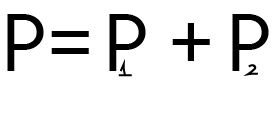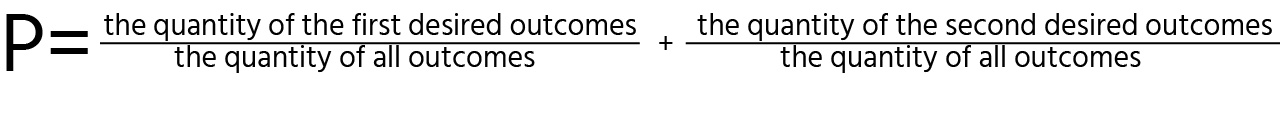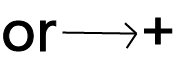Course Content

Probability TheoryAdd Probabilities

Probability theory can describe a many situations in our lives; for that reason, learning it should be interesting!

Where can I use it?

In situations when either one of the events should happen. Events should be absolutely independent.

Example:

At the beginning of the course, we acquinted ourselves with the definition of probability theory with the example of balls. But now, this example will be complicated.

Calculate the probability of getting either a green or blue ball if you have `9` blue balls, `13` red balls, and `7` green balls.Explanation:

So, to calculate the probability of success for the events connected by the word or (get the blue or green ball from the box), we should calculate the probability for the first event, then add the probability for the second event, and so on. We can use this schema for calculating three, four, or even a million possibilities, and just calculate the probability for each of them, and finally add.

#Calculate the probability of winning a lottery with the \$15 000 or \$10 000 ticket. The probability of pulling a \$15 000 lottery winning ticket is 25%. The probability of pulling a \$10 000 lottery winning ticket is 15%. Replace ___ with it.

`%`Section 2.

Chapter 1